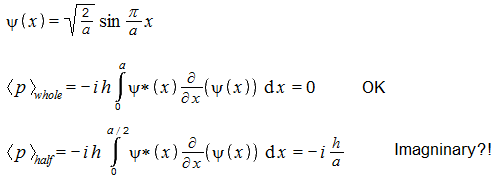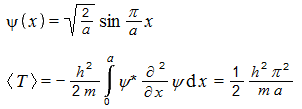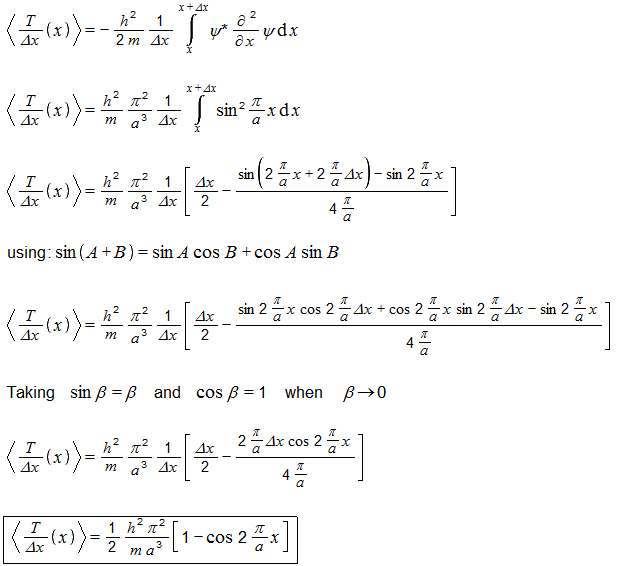## Recommended Posts

Hello... Say there is a particle in a 1D box. The particle is in certain state. I know its position-space wave function.

I can compute expectation value of its momentum by sandwiching the momentum operator between psi* and psi and integrating over the whole box width (or over whole infinity).

Question... If I don't integrate over the whole box, but only over a part of the box, can the result be interpreted as follows: if I simultaneously measure momentum and position of identically prepared setups, and if I only consider those cases where a particle was found within the part of the box for which I computed the integral, then the average momentum of those particles will equal to my integral?

##### Share on other sites
44 minutes ago, Danijel Gorupec said:

and if I only consider those cases where a particle was found within the part of the box for which I computed the integral,

##### Share on other sites
1 hour ago, studiot said:

So, from various sources this is an time-independent wave-function for a ground state particle in an infinite square (widht 'a'). The momentum calculation gives zero, as expected.But if I only integrate over half of the box width (the last line) then I get an imaginary result. Does this mean that this result has no interpretation (that it, it means nothing)?

[sorry, the 'h' should actually be h-bar, but I don't have this symbol]

Edited by Danijel Gorupec

##### Share on other sites

Hmm... but let's compute expectation value for kinetic energy... I am doing this for the same wave-function (ground state particle-in-a-box):

('h' is actually h-bar)The above is the correct result when the integral is computed over the whole box. But even if I integrate over only a part of the box, I still obtain real values - does it mean that such result might have some actual meaning?

Not being lazy, I tried to compute kinetic energy distribution (or density) over the box. Moderators can move this to the speculation forum.

I am using a sloppy notation as I know no better.This is the kinetic energy density function for a ground-state particle in a 1D box, how I calculated it.... Can this have any meaning? The function shape resembles the position probability density function for the particle.

I would be very glad if there is any function that can show kinetic energy density for wave-like particles. I like energy distributed in space (that is, local i like the local conservation of it). However, I never heard anything about it.

##### Share on other sites

What is the point of doing the integral over only part of the spatial extent?

##### Share on other sites
On 11/11/2019 at 2:05 AM, swansont said:

What is the point of doing the integral over only part of the spatial extent?

This is what I actually wonder - does a quantity obtained that way has any known meaning?

For the momentum I obtain imaginary results which suggest it does not have a meaning.

For kinetic energy I obtain real result. It might have a meaning. I used this to derive something that seems to me as the 'kinetic energy density' function. But I never heard of such thing before.

##### Share on other sites
2 hours ago, Danijel Gorupec said:

This is what I actually wonder - does a quantity obtained that way has any known meaning?

Of course it has a meaning.

your integral represent the probability of finding the particle in that part of the box defined by your limits of integration.

So when normalised this should be 1/2 for half the box and so on.

Just as the integral formula you are summing is the probability of finding the particle between x and (x+dx) (or in some cases between (x-dx/2) and (x+dx/2)

But as swansont says

Of what use is it?

Any interaction needs must involve the whole box.

##### Share on other sites

Hi Danijel and the others,

you wrote the dependence of Psi on the position but did not write the dependence on time.

When the wavefunction is stationary, that is if |Psi|2 is independent on time, it has still a multiplicative term exp (j2piEt/h). On the probability density of a stationary wave the term disappears, but on the momentum it doesn't.

With this time term, <p> is not just a constant number, imaginary or not. Its phase rotates over time with frequency E/h.

Whether <p> is imaginary at t=0 depends on your arbitrary choice of time origin. Drift the time convention by E/4h and an imaginary value at t=0 gets real.

So the imaginary value you got does not mean a value to be discarded. More generally, complex numbers are excellent to describe sine amplitudes: their two components just describe the in-phase and in-quadrature components of a sine, both being very tangible when the wave is.

==========

By the way, if you write this term of time dependence in a p orbital, you can compute how quickly a plane of constant phase rotates around the nucleus, and relate this simply with the orbital angular momentum of the electron. Then, the orbital angular momentum is nicely concrete and similar to the macroscopic understanding - except that the electron shows no bulge moving around the nucleus.

Through the phase of the complex wavefunction, QM explains why the electron has an orbital angular momentum but the probability distribution has no movement that would radiate light. Some attributes of a movement, but not all.

You can also compute the movement of equiphase planes for the plane wavefunction of a massive particle, and compare with the particle's momentum and speed. This will tell you why the ancestors defined the momentum operator that way. And do the same with the kinetic energy.

==========

Computing the kinetic energy over a part of the spatial extent does have uses and is legitimate.

For instance to compute how the relativistic mass changes the electron's energy levels in a hydrogen or hydrogen-like (one electron, several protons) atom. The mass correction must be done locally, not once over the whole extension, to get the experimental result.

Or if an other particle interacts with the electron, and that other particle is concentrated over a portion of the electron's extension, it will feel the attributes the electron has in that region.

Edited by Enthalpy

##### Share on other sites

Sorry I didn't see something all-important.

sin (pi*x/a) is not a proper function (eigenfunction) of the momentum operator. That's why the computed "measure" gives a fluctuating value.

This sin () is the difference (with normalisation) of two functions:
exp (j*pi*x/a) and exp (-j*pi*x/a)
each is a proper function and gives a constant computed momentum.

That is:

• A proper functions for the energy, here sin (pi*x/a), is not necessarily one for the momentum;
• A linear combination of two proper functions isn't necessarily one. When both have the same proper value, yes. If not, often (always?) no. Here the momentums are opposite;
• A physical measurement of the momentum would give either one value or the other;
• The computed measure for sin () is little useful.

Fun too: the particle is localized in the box but its momentum seems exactly known. Though, the momentum can have two values, towards +X and -X, which gives uncertainty. I didn't check the figures. A similar situation appears with electrons localized on a ring in simple models for benzene.

## Create an account

Register a new account